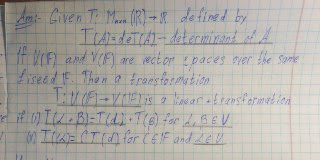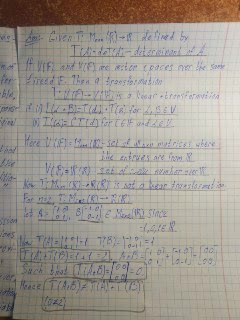gamau6v

2021-11-17

Determine whether or not the following transformation T : V \rightarrow W is a linear transformation. If T is not a linear transformation, provide a counter example. If it is, then: (i) find the nullspace N(T) and nullity of T, (ii) find the range R(T) and rank of T , (iii) determine if T is one-to-one, (iv) determine if T is onto.juniorekze

Step 1Step 2Do you have a similar question?